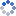加载中…大仙• 博客等级：• 博客积分：0
• 博客访问：22,200,989
• 关注人气：15,895
• 获赠金笔：0支
• 赠出金笔：0支
• 荣誉徽章：

## 中国奥运七剑下金牌(2008-08-26 03:29:37)
 分类： 大仙新浪体育评论

总金 163（15+05+16+16+28+32+51）
男子 71 （10+02+04+07+11.5+12.5+24）
女子 92 （05+03+12+09+16.5+19.5+27）

跳水   27 （1+2+3+3+5+6+7）
举重   24 （4+0+0+2+5+5+8）
体操   22 （5+1+2+1+3+1+9）
乒乓球 20 （2+3+4+4+3+4）
射击   19 （3+0+2+2+3+4+5）
羽毛球 11 （0+1+4+3+3）
柔道   08 （0+0+1+1+2+1+3）
游泳   07 （0+0+4+1+0+1+1）
田径   05 （0+0+1+1+1+2+0）
跆拳道 04 （1+2+1）
女排   02 （1+0+0+0+0+1+0）
击剑   02 （1+0+0+0+0+0+1）
皮划艇 02 （0+0+0+0+0+1+1）
摔跤   02 （0+0+0+0+0+1+1）
拳击   02 （0+0+0+0+0+0+2）
蹦床   02 （0+2）
网球   01 （0+0+0+0+0+1+0）
射箭   01 （0+0+0+0+0+0+1）
赛艇   01 （0+0+0+0+0+0+1）
帆板   01 （0+0+0+0+0+0+1）

至今无金项目：足球（男足、女足）、篮球（男篮、女篮）、男排、手球（男子、女子）、水球（男子、女子）、曲棍球（男子、女子）、棒球、垒球、自行车、马术、现代五项、铁人三项、花样游泳、艺术体操、帆船、10公里马拉松游泳；细分则有男子游泳、男子网球、男子射箭、男子柔道、男子跆拳道、男子摔跤、男子赛艇、女子皮划艇。

可以看出，中国跳水是当之无愧的梦之队，领衔中国体育奥运夺金的第一集团。只有四个比赛项目、并且1988年汉城奥运会才开始设立的乒乓球，以国球的姿态为中国统治20枚金牌。跳水和体操两大梦幻军团，参加七届，七届夺金，一届不落。而举重、射击、柔道和羽毛球，也是中国体育固守奥运金牌的重镇。体操、举重、跳水、射击、乒乓球、羽毛球、柔道在北京奥运会一共为中国获得39枚金牌，这七大强项就足以超过美国所取得的36枚金牌。
所以，从中国竞技体育“奥运战略”也就是“金牌战略”的发展路线来看，国家一定会重点投入七大奥运强项，营造“七剑下金牌
”的声势，这七大强项为——乒乓球、羽毛球、跳水、体操、举重、射击、柔道；其他11个具有一定影响力的项目——篮球、排球、田径、游泳、击剑、射箭、摔跤、赛艇、帆船、速度滑冰、短道速度滑冰，国家也比较热心投入；而对足球、网球、手球、曲棍球、棒球、垒球、水球、花样游泳、艺术体操、拳击、自行车、皮划艇、现代五项、马术、武术、花样滑冰、冰球、越野滑雪、冬季两项、跳台滑雪、自由式滑雪这些相对落后或影响不大的项目，国家只做限量投入。

（本文供新浪奥运博客独家刊登，其它网纸媒体请勿采用）

0